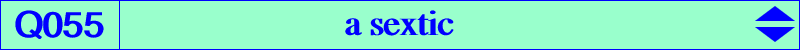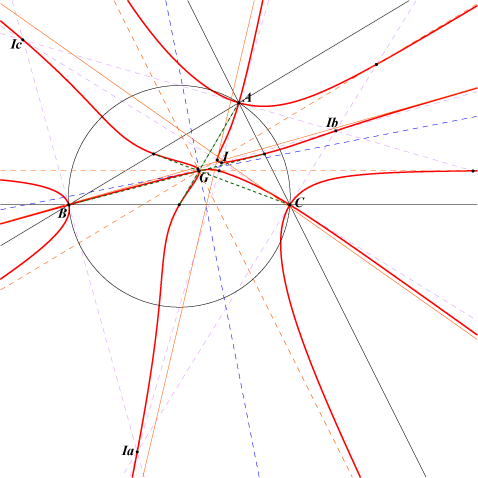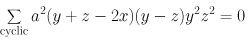X(1), X(2) midpoints of ABC excenters seven central cyclocevian points, see table 24. other points belowLet M be a point and MaMbMc its cevian triangle. Denote by C(M) the conic with center M passing through Ma, Mb, Mc and by I(M) the in-conic with center M. These two conics are bitangent at two points lying on the conjugated diameter of the trilinear polar of M with respect to both conics. Thus C(M) and I(M) generate a pencil of bitangent conics which contains a circle if and only if M lies on Q055. Note that the pencil also contains a rectangular hyperbola. Q055 contains four nodes : G (with two tangents parallel to the asymptotes of the Kiepert hyperbola) and A, B, C (the tangents are the bisectors of ABC). Q055 has six real asymptotes : three are parallel to those of the Thomson cubic and three are the parallel at G to the sidelines of ABC. These latter asymptotes meet the sextic again on the bisectors of ABC. Q055 contains the midpoints of ABC and the tangents at these points are the medians of ABC. For example, with M = I, (M) is the conic passing through X(244), X(2170), X(2446), X(2447), X(2611) circumscribing the incentral triangle and (m) is the incircle. See also Table 24.